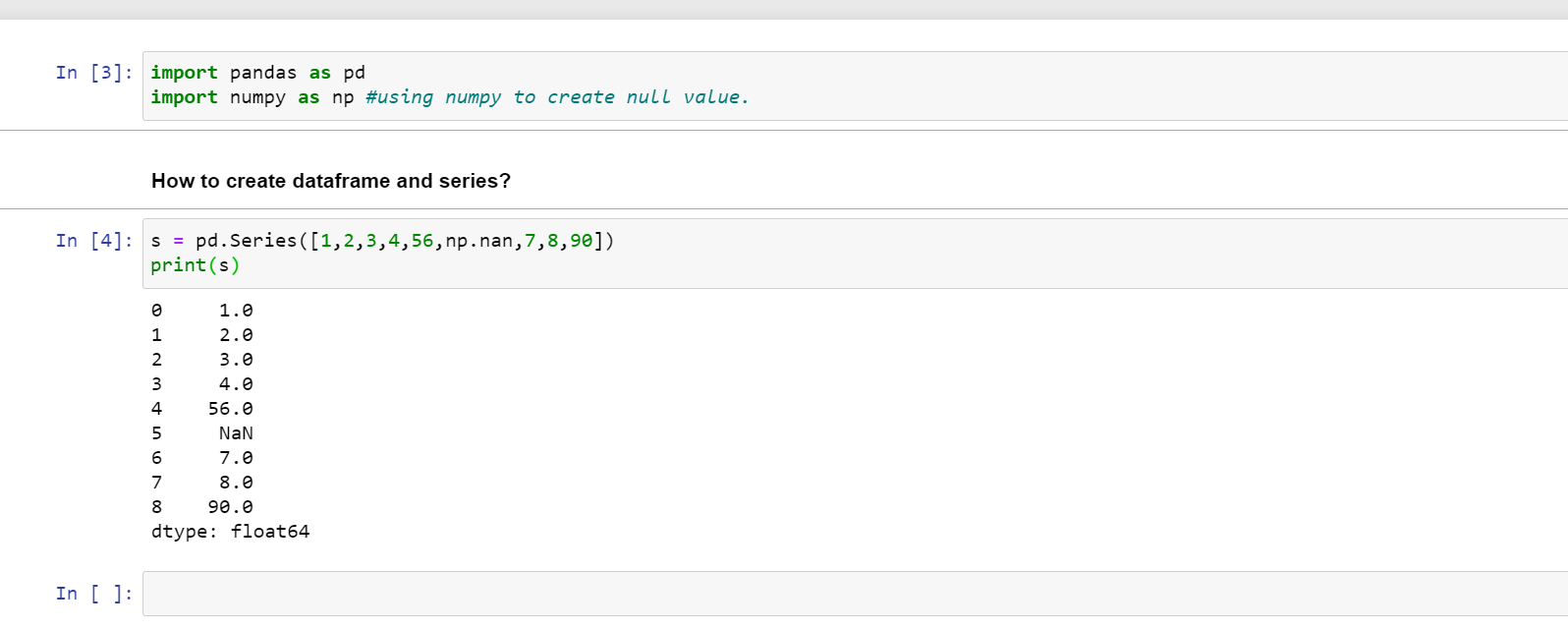1602926340

# Pandas Dtype-Specific Operations: Accessors

Note: All images created by author unless stated otherwise.

Pandas is a widely-used data analysis and manipulation library in Python. It provides numerous functions and methods to work with any type of data. There are also methods that work only with a specific data type. These methods are accessed through 4 accessors.

The accessors extend the capabilities of Pandas and provide specific operations. For instance, extracting the month from the date can be done using the dt accessor.

In this post, we will see various operations with 4 accessors of Pandas which are:

• Str: String data type
• Cat: Categorical data type
• Dt: Datetime, Timedelta, Period data types
• Sparse: Sparse data type

Note: We will work the examples on Pandas Series which can also be considered as DataFrame columns.

#artificial-intelligence #data-analysis #machine-learning #pandas #data-science

## Buddha Community1619565060

## Ternary operator in Python?

1. Ternary Operator in Python

What is a ternary operator: The ternary operator is a conditional expression that means this is a comparison operator and results come on a true or false condition and it is the shortest way to writing an if-else statement. It is a condition in a single line replacing the multiline if-else code.

syntax : condition ? value_if_true : value_if_false

condition: A boolean expression evaluates true or false

value_if_true: a value to be assigned if the expression is evaluated to true.

value_if_false: A value to be assigned if the expression is evaluated to false.

How to use ternary operator in python here are some examples of Python ternary operator if-else.

Brief description of examples we have to take two variables a and b. The value of a is 10 and b is 20. find the minimum number using a ternary operator with one line of code. ( **min = a if a < b else b ) **. if a less than b then print a otherwise print b and second examples are the same as first and the third example is check number is even or odd.

#python #python ternary operator #ternary operator #ternary operator in if-else #ternary operator in python #ternary operator with dict #ternary operator with lambda1598629440

## How To Perform Set Operations On Pandas DataFrames

In this article we will explore Pandas Set operations that are Union, Intersection and Difference and will see how to use them?

#developers corner #pandas #pandas dataframes #pandas set operation

1586702221

## Python Pandas Objects - Pandas Series and Pandas Dataframe

In this post, we will learn about pandas’ data structures/objects. Pandas provide two type of data structures:-

### Pandas Series

Pandas Series is a one dimensional indexed data, which can hold datatypes like integer, string, boolean, float, python object etc. A Pandas Series can hold only one data type at a time. The axis label of the data is called the index of the series. The labels need not to be unique but must be a hashable type. The index of the series can be integer, string and even time-series data. In general, Pandas Series is nothing but a column of an excel sheet with row index being the index of the series.

### Pandas Dataframe

Pandas dataframe is a primary data structure of pandas. Pandas dataframe is a two-dimensional size mutable array with both flexible row indices and flexible column names. In general, it is just like an excel sheet or SQL table. It can also be seen as a python’s dict-like container for series objects.

#python #python-pandas #pandas-dataframe #pandas-series #pandas-tutorial1602926340

## Pandas Dtype-Specific Operations: Accessors

Note: All images created by author unless stated otherwise.

Pandas is a widely-used data analysis and manipulation library in Python. It provides numerous functions and methods to work with any type of data. There are also methods that work only with a specific data type. These methods are accessed through 4 accessors.

The accessors extend the capabilities of Pandas and provide specific operations. For instance, extracting the month from the date can be done using the dt accessor.

In this post, we will see various operations with 4 accessors of Pandas which are:

• Str: String data type
• Cat: Categorical data type
• Dt: Datetime, Timedelta, Period data types
• Sparse: Sparse data type

Note: We will work the examples on Pandas Series which can also be considered as DataFrame columns.

#artificial-intelligence #data-analysis #machine-learning #pandas #data-science1602550800

## Pandas in Python

Pandas is used for data manipulation, analysis and cleaning.

What are Data Frames and Series?

Dataframe is a two dimensional, size mutable, potentially heterogeneous tabular data.

It contains rows and columns, arithmetic operations can be applied on both rows and columns.

Series is a one dimensional label array capable of holding data of any type. It can be integer, float, string, python objects etc. Panda series is nothing but a column in an excel sheet.

### How to create dataframe and series?

s = pd.Series([1,2,3,4,56,np.nan,7,8,90])

print(s)How to create a dataframe by passing a numpy array?

1. d= pd.date_range(‘20200809’,periods=15)
2. print(d)
3. df = pd.DataFrame(np.random.randn(15,4), index= d, columns = [‘A’,’B’,’C’,’D’])
4. print(df)

#pandas-series #pandas #pandas-in-python #pandas-dataframe #python